# The graph of the function y = x 3 without using a calculator.### Single Variable Calculus: Concepts...

4th Edition
James Stewart
Publisher: Cengage Learning
ISBN: 9781337687805### Single Variable Calculus: Concepts...

4th Edition
James Stewart
Publisher: Cengage Learning
ISBN: 9781337687805

#### Solutions

Chapter T, Problem 5CDT

a)

To determine

## To sketch: The graph of the function y=x3 without using a calculator.

Expert Solution

### Explanation of Solution

Compute a table for various values of x and then use the value of the function to sketch a smooth curve of y=x3.

 x −2 −1 0 1 2 y −8 −1 0 1 8

The graph for the given function y=x3 is shown below in Figure 1.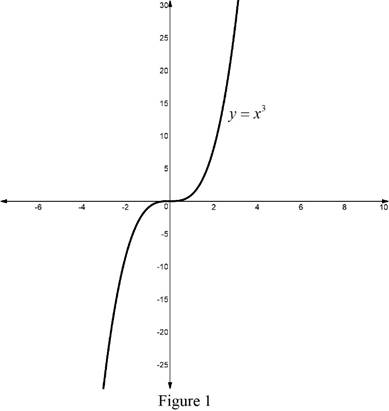From Figure 1 it is observed that, the graph of the function y=x3 is unbounded region.

b)

To determine

Expert Solution

### Explanation of Solution

Compute a table for various values of x and then use the value of the function to sketch a smooth curve of y=(x+1)3.

 x −2 −1 0 1 2 y −1 0 1 8 27

The graph for the given function y=(x+1)3 is shown below in Figure 2.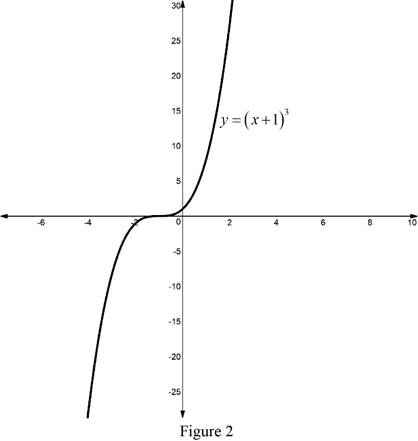From Figure 2 it is observed that, the graph of the function y=(x+1)3 is unbounded region. The graph of the function y=(x+1)3 is translated right by 1 units.

c)

To determine

Expert Solution

### Explanation of Solution

Compute a table for various values of x and then use the value of the function to sketch a smooth curve of y=(x2)3.

 x −2 −1 0 1 2 y −64 −27 −8 −1 0

The graph for the given function y=(x2)3 is shown below in Figure 3.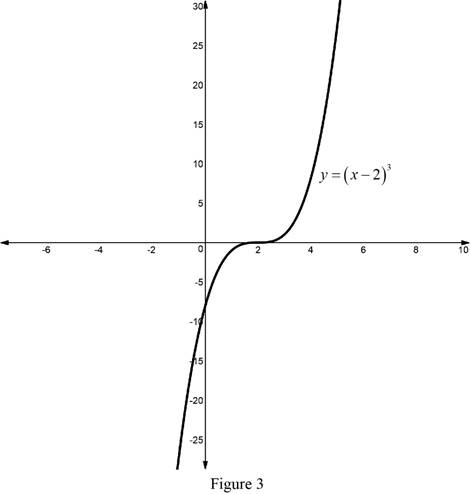According to the transaltion and reflection of the function the graph of the function y=(x2)3 is obtained if the graph of y=(x2)3 is translated upward by 3 units.

The graph for the given function y=(x2)3+3 is shown below in Figure 4.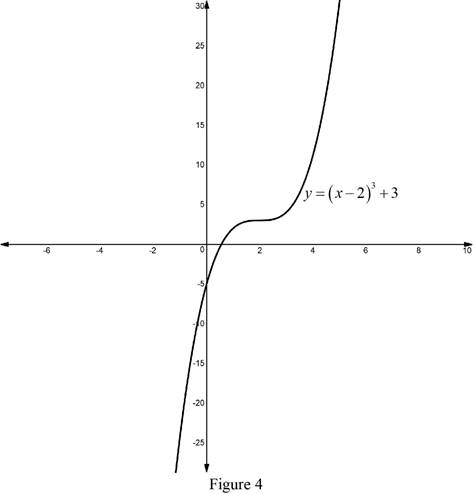From Figure 2 , it is observed that the graph of the function y=(x2)3+3 is obtained if the graph of the function y=(x2)3+3 is translated right by 2 units and upward by 3 units.

d)

To determine

Expert Solution

### Explanation of Solution

Compute a table for various values of x and then use the value of the function to sketch a smooth curve of y=x2.

 x −2 −1 0 1 2 y 4 1 0 1 4

The graph for the given function y=x2 is shown below in Figure 5.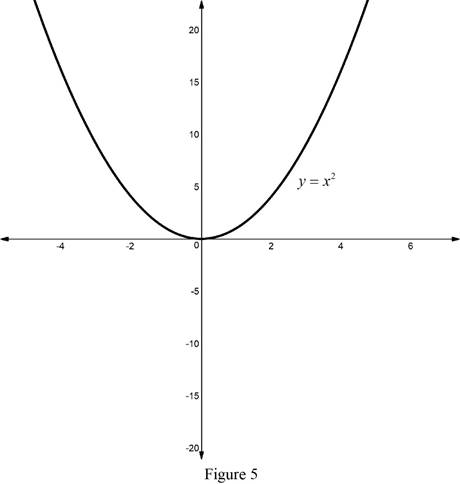According to the transaltion and reflection of the function the graph of the function y=x2 is obtained if the graph of y=x2 is translated upward by 4 units.

The graph for the given function y=4x2 is shown below in Figure 6.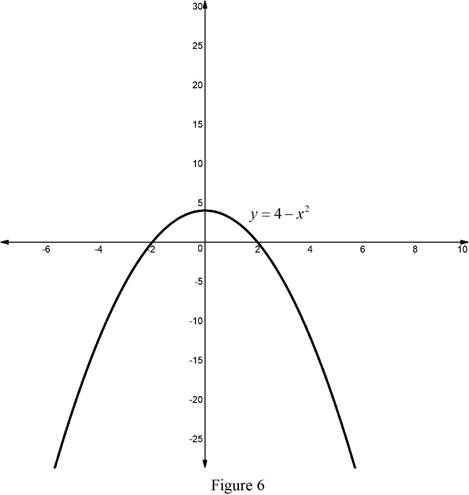From Figure 6 it is observed that, the graph of the function y=4x2 is unbounded region.

e)

To determine

Expert Solution

### Explanation of Solution

Compute a table for various values of x and then use the value of the function to sketch a smooth curve of y=x.

 x 1 2 3 4 5 y 1 2 3 2 5

The graph for the given function y=x is shown below in Figure 7.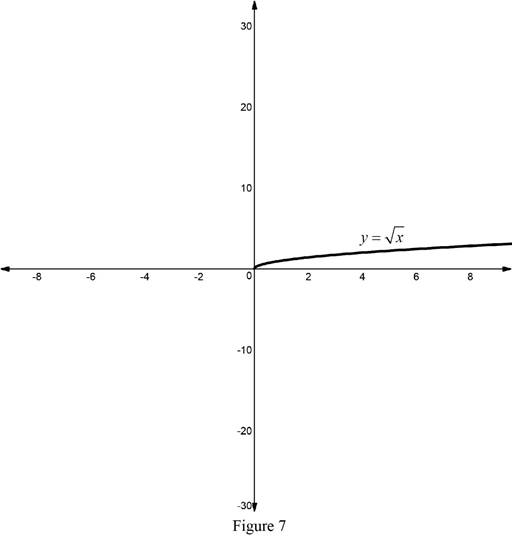From Figure 7 it is observed that, the graph of the function y=x is unbounded region.

f)

To determine

Expert Solution

### Explanation of Solution

Compute a table for various values of x and then use the value of the function to sketch a smooth curve of y=2x.

 x 1 2 3 4 5 y 2 22 23 4 25

The graph for the given function y=2x is shown below in Figure 8.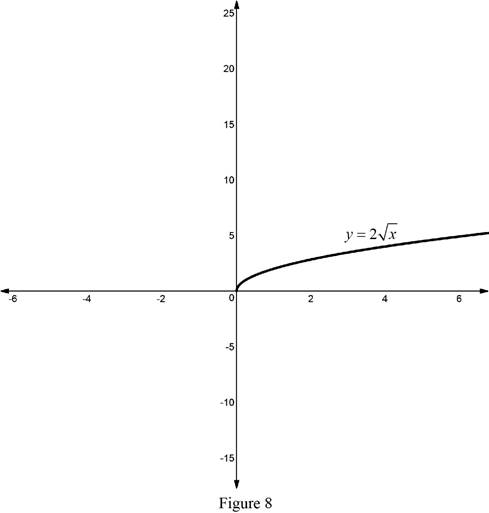From Figure 8 it is observed that, the graph of the function y=2x is unbounded region.

g)

To determine

Expert Solution

### Explanation of Solution

Compute a table for various values of x and then use the value of the function to sketch a smooth curve of y=2x.

 x −1 0 1 2 3 y 12 1 2 4 8

The graph for the given function y=2x is shown below in Figure 9.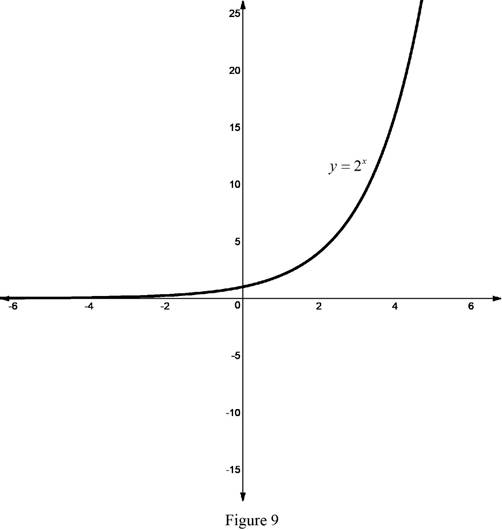Reflect the graph of y=2x about the x axis then obtained y=2x. The graph for the given function y=2x is shown below in Figure 10.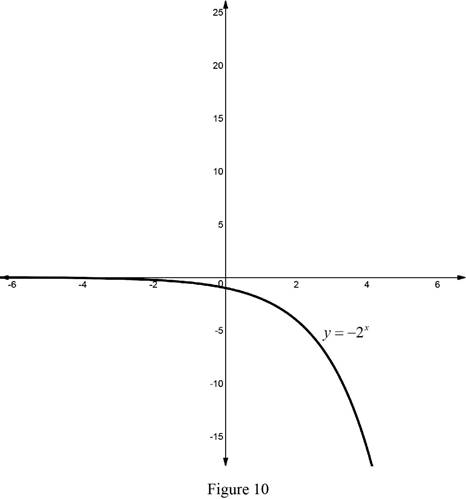From Figure 10 it is observed that, the graph of the function y=2x is unbounded region.

g)

To determine

Expert Solution

### Explanation of Solution

Compute a table for various values of x and then use the value of the function to sketch a smooth curve of y=x1.

 x −12 1 12 −13 −14 y −2 1 2 −3 −4

The graph for the given function y=x1 is shown below in Figure 11.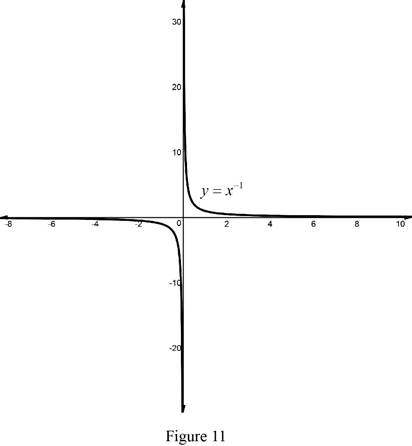According to the translation of the function the graph of the function y=x1 is obtained if the graph of y=x1 is translated upward by 1 units.

The graph for the given function y=1+x1 is shown below in Figure 12.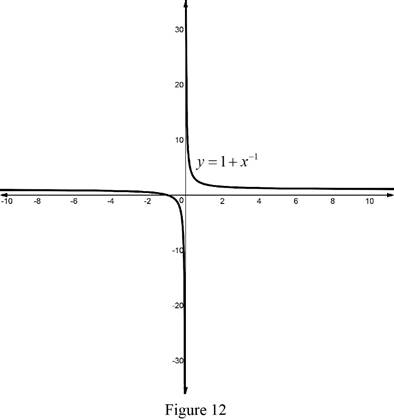From Figure 12 it is observed that, the graph of the function y=1+x1 is unbounded region.

### Have a homework question?

Subscribe to bartleby learn! Ask subject matter experts 30 homework questions each month. Plus, you’ll have access to millions of step-by-step textbook answers!